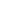Circles GEO-1008 is a Course

Circles GEO-1008

Self-paced

Full course description

Course Description

You see circles every day, from the tires on your car, to the rim of your coffee mug. This course covers circles, including how to calculate the area of a circle.

By the end of this course, you will be able to

• Define a circle

• Define terms related to circles, including radius, chord, and diameter

• Measure arcs

• Define pi

• Calculate the circumference of a circle

• Calculate the length of an arc

• Calculate the area of a circle

• Calculate the area of a sector

Estimated completion time (hours): 0.9